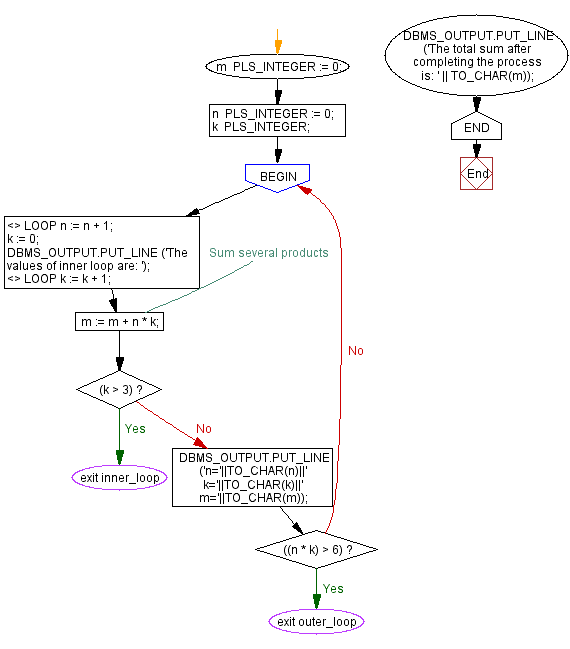﻿ PL/SQL Control Statement: Show the uses of nested loop - w3resource# PL/SQL Control Statement Exercises: Show the uses of nested loop

## PL/SQL Control Statement: Exercise-18 with Solution

Write a program in PL/SQL to show the uses of nested loop.

Sample Solution:

PL/SQL Code:

``````DECLARE
m  PLS_INTEGER := 0;
n  PLS_INTEGER := 0;
k  PLS_INTEGER;
BEGIN
<>
LOOP
n := n + 1;
k := 0;
DBMS_OUTPUT.PUT_LINE ('The values of inner loop are: ');
<>
LOOP
k := k + 1;
m := m + n * k; -- Sum several products

EXIT inner_loop WHEN (k > 3);
DBMS_OUTPUT.PUT_LINE ('n='||TO_CHAR(n)||'  k='||TO_CHAR(k)||'  m='||TO_CHAR(m));
EXIT outer_loop WHEN ((n * k) > 6);
END LOOP inner_loop;
END LOOP outer_loop;
DBMS_OUTPUT.PUT_LINE
('The total sum after completing the process is: ' || TO_CHAR(m));
END;
/
```
```

Flowchart:Sample Output:

```SQL> /
The values of inner loop are:
n=1  k=1  m=1
n=1  k=2  m=3
n=1  k=3  m=6
The values of inner loop are:
n=2  k=1  m=12
n=2  k=2  m=16
n=2  k=3  m=22
The values of inner loop are:
n=3  k=1  m=33
n=3  k=2  m=39
n=3  k=3  m=48
The total sum after completing the process is: 48

PL/SQL procedure successfully completed.```

Improve this sample solution and post your code through Disqus

What is the difficulty level of this exercise?

﻿